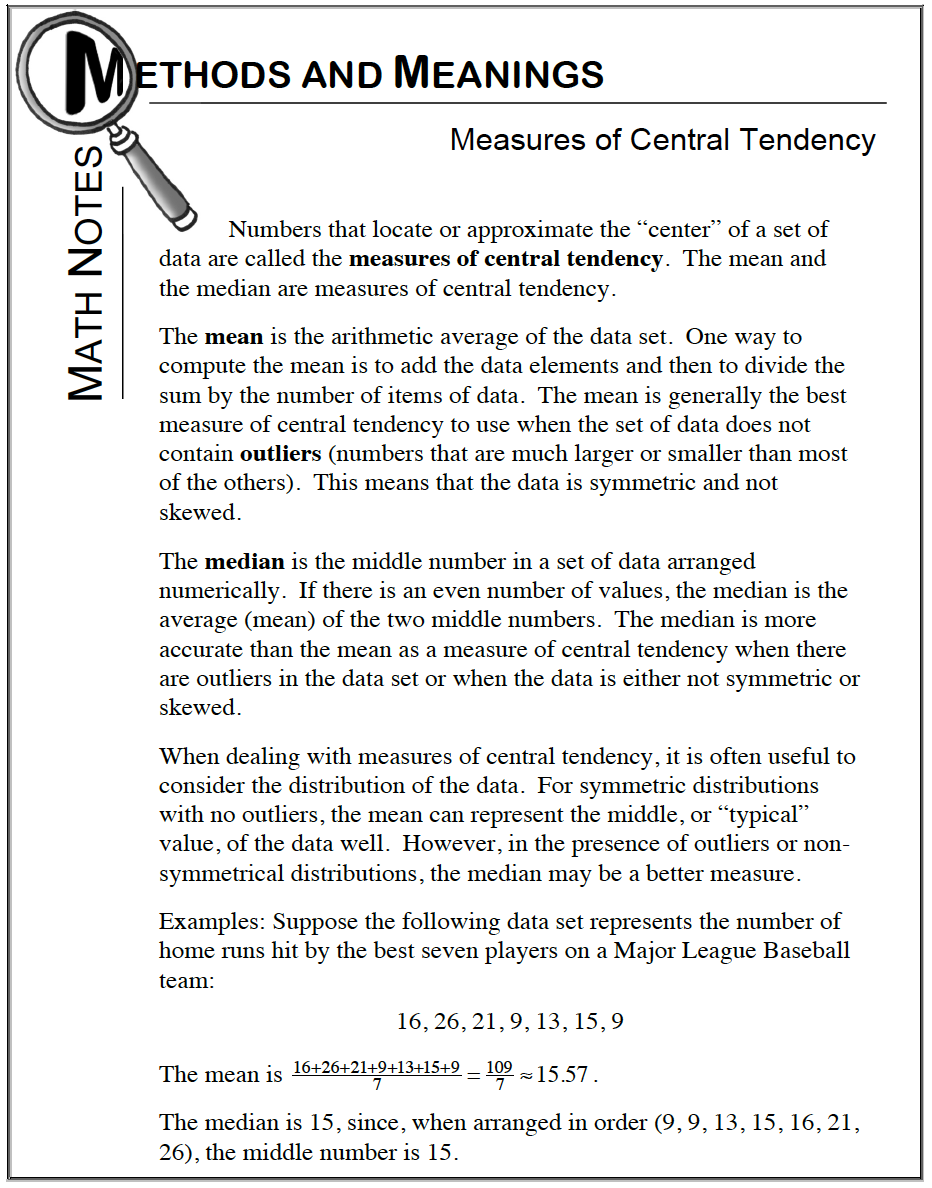### Home > CC2 > Chapter 3 > Lesson 3.2.4 > Problem3-76

3-76.

Find the mean, range, and median of the values: $12$, $4$, $-2$, $0$, $9$, $-2$, $1$, $7$, $8$, $2$. Recall that the range is calculated by finding the difference between the largest and smallest data values. Homework Help ✎

The range of a set of data is the difference between the highest and the lowest values.

Refer to the Math Notes box below.